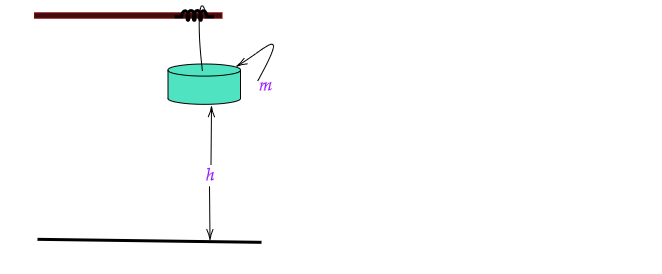# Derive the formula for potential energy.

Potential Energy: Energy of a body or object caused by its position is known Potential Energy. It is also called Mechanical Energy or Fixed energy. It is denoted by $P.E.$ or $P$. In S.I. its unit is Joule.

Derivation of the Potential Energy formula:Let In the given figure, the body of mass $m$ is placed on the height of $h$.

Then the work done to place the body on the height $h$,

$W=F\times h$

Here $F$ is the gravitational force applied against the gravity and $F=mg$

Therefore, Work done to place the body form the ground to the height $h$

$=mgh$

And the work done locate the body to the height $h$ is the Potential Energy  or Stored enrgy.

Thus, Potential Energy $\boxed{P.E.=mgh}$

Updated on: 10-Oct-2022

425 Views